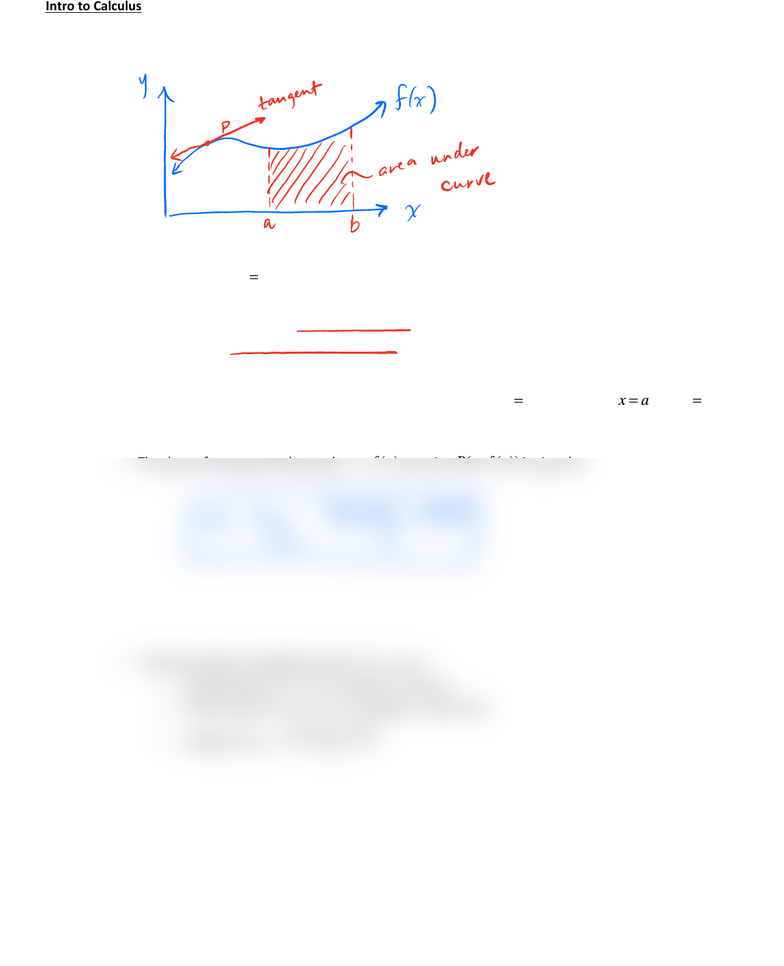# MAT135H1 Lecture Notes - Lecture 1: Differential Calculus

14 views2 pagesIntro to Calculus
What is Calculus?
o Two simple geometric problems:
o Given a function
()
yfx
=
,
! The problem of tangents [differential calculus]
What is the slope of the tangent to the graph of the function at a given pointP’? (i.e.
instantaneous rate of change)
! The problem of areas [integral calculus]
What is the area under the graph of a function
()
yfx
=
between
xa
=
and
xb
=
?
o The slope of a tangent to the graph
()
yfx
=
at point
(, ())
Pa f a
is given by:
o To find the slope of a tangent at point
(, ())
Pa f a
! find the value of
()
fa
[i.e. replace x with a]
! find the value of
fa h
+
[i.e. replace x with a+h]
! evaluate 0
()()
limh
fa h fa
h
+
I
mlijnoHath fCa
Unlock document

This preview shows half of the first page of the document.
Unlock all 2 pages and 3 million more documents.